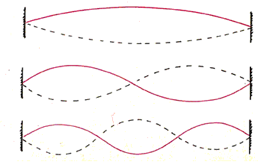# Big Standing Wave - Small Effort!

A standing wave is formed when two identical traveling waves continually pass through the same medium in opposite directions. One convenient way to produce standing transverse waves is to allow a traveling transverse wave sent continuously down the Super Springy to interfere with its own reflection.  To do this, the spring should be stretched out a specified distance and held firmly in place at the far end.  The spring is moved rapidly in a back and forth motion in order to produce continuous transverse pulse down the spring. The reflected pulses are inverted (i.e., "shifted" one-half wavelength). This causes a node (result of total destructive interference) to form at the far end of the spring, and allows for anti-nodes, or loops, to be formed along the length of the spring.

In the video, you see the Super Springy stretched out a distance of 24 feet. By adjusting the frequency of the waves, the wavelength may be manipulated so that different numbers of loops can be formed. As with all standing waves, the length of one loop is one-half wavelength. You can find the wavelength (λ) of the standing wave by dividing its total length by the number of loops to get the length of one loop, and then doubling it. Since the Super Springy is stretched out 24 feet, the wavelength when two loops are formed will be 24 feet, the wavelength when 3 loops are formed will be 16 feet, and when four lops are formed will be 12 feet, and for 5 loops, the wavelength will be 2/5 of 24 feet = 9.6 feet.You can determine the frequency of the waves by timing my motion for 10 complete cycles. The frequency in Hertz will be 10 cycles divided by the time in seconds taken to produce the 10 cycles. You can then multiply the frequency of the standing wave by its wavelength to determine the speed of the wave in the Super Springy. Since the tension in the Springy remained constant for all trials, you should expect to calculate the same speed for the wave, regardless of the number of loops formed.

In the video clips, the total time needed to produce 10 cycles when 2 loops were formed was approximately 9.75 sec. This results in a frequency f of 10 cycles / 9.75 sec = 1.026 Hz. Since the wavelength λ is 24 feet, the speed v of the wave in the Super Springy is calculated as v = f λ = 1.026 Hz x 24 feet = 24.62 ft/s.

Similarly, the frequency when 3 loops were formed = 10 cycles / 6.21 s = 1.610 Hz. The speed of the wave when 3 loops were formed is v = f λ = 1.610 Hz x 16 feet = 25.76 ft/s.

For 4 loops, the frequency is found to be 2.16 Hz (10 cycles in 4.64 seconds), yielding a speed v of v = f λ = 2.16 Hz x 12 feet = 25.92 ft/s. The frequency for 5 loops was 2.75 Hz (10 cycles in 3.64 sec), resulting in a wave speed of v = f λ = 2.75 Hz x 9.6 feet = 26.40 ft/s.

*Slight variations in the calculated speeds can likely be attributed to errors in measuring time and quite likely in not producing the most perfect standing wave form.

It should be no surprise that the speed calculations for standing wave are approximately equal (average speed = 25.68 ft/s), since the speed of a wave is determined by the properties of the medium, and is not affected by changes in the frequency or amplitude of the generated wave. In this case, since all standing waves were formed in same large spring that was maintained at the same constant tension, all waves should have the same speed. The actual speeds your students measure when they perform this investigation for themselves will depend on the type of spring used and how tightly it is stretched out. Students can check their wave speed by timing a single pulse as it travels down the length of the spring and back and dividing the total distance traveled by the total time.

The free computer simulation at http://phet.colorado.edu/en/simulation/wave-on-a-string allows you to further investigate wave motion, including standing waves, on a "virtual" spring.  You can read more about the production of standing waves at the free web site http://www.physicsclassroom.com/Class/waves/u10l4a.cfm.

Collin Wassilak